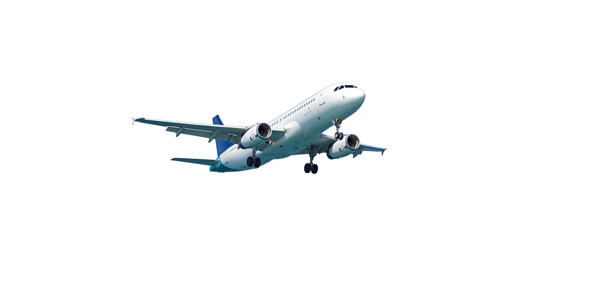# Phoenix Va S340 Type Rating - Aircraft Exam

10 Questions | Total Attempts: 38SettingsExam required for Saab 340 type rating at Phoenix Virtual Airlines.

• 1.
What is the country of origin of the Saab 340?
• A.

Germany

• B.

Sweden

• C.

France

• D.

• 2.
What is the commercial service ceiling of the S340?
• A.

FL180

• B.

FL230

• C.

FL250

• D.

FL290

• 3.
Which is the closest to the empty weight of the S340?
• A.

17,700 lbs

• B.

18,000 lbs

• C.

19,450 lbs

• D.

19,700 lbs

• 4.
What is the wingspan of the S340?
• A.

17.05m

• B.

18.22m

• C.

20.10m

• D.

21.44m

• 5.
How many blades are on each prop?
• A.

3

• B.

4

• C.

5

• D.

6

• 6.
The maximum loaded weight is 27,002 lbs?
• A.

True

• B.

False

• 7.
The powerplant of the S340 is two General Electric CT7-9B turboprops rated at 1295 shp.
• A.

True

• B.

False

• 8.
Which model introduced vibration control?
• A.

S340 A

• B.

S340 B

• C.

S340 B Plus

• D.

S340 QC

• 9.
What is the maximum speed?
• A.

276 kts

• B.

282 kts

• C.

291 kts

• D.

301 kts

• 10.
What is the minimum runway length at maximum takeoff weight?
• A.

1000m

• B.

1300m

• C.

1450m

• D.

1600m

Related TopicsBack to top